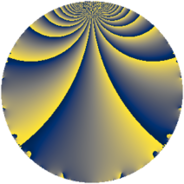# Properties

 Label 574.2.aLevel $574$ Weight $2$ Character orbit 574.a Rep. character $\chi_{574}(1,\cdot)$ Character field $\Q$ Dimension $19$ Newform subspaces $13$ Sturm bound $168$ Trace bound $5$

# Related objects

## Defining parameters

 Level: $$N$$ $$=$$ $$574 = 2 \cdot 7 \cdot 41$$ Weight: $$k$$ $$=$$ $$2$$ Character orbit: $$[\chi]$$ $$=$$ 574.a (trivial) Character field: $$\Q$$ Newform subspaces: $$13$$ Sturm bound: $$168$$ Trace bound: $$5$$ Distinguishing $$T_p$$: $$3$$, $$5$$, $$11$$

## Dimensions

The following table gives the dimensions of various subspaces of $$M_{2}(\Gamma_0(574))$$.

Total New Old
Modular forms 88 19 69
Cusp forms 81 19 62
Eisenstein series 7 0 7

The following table gives the dimensions of the cuspidal new subspaces with specified eigenvalues for the Atkin-Lehner operators and the Fricke involution.

$$2$$$$7$$$$41$$FrickeDim.
$$+$$$$+$$$$+$$$$+$$$$2$$
$$+$$$$+$$$$-$$$$-$$$$4$$
$$+$$$$-$$$$+$$$$-$$$$3$$
$$+$$$$-$$$$-$$$$+$$$$1$$
$$-$$$$+$$$$+$$$$-$$$$3$$
$$-$$$$+$$$$-$$$$+$$$$1$$
$$-$$$$-$$$$+$$$$+$$$$2$$
$$-$$$$-$$$$-$$$$-$$$$3$$
Plus space$$+$$$$6$$
Minus space$$-$$$$13$$

## Trace form

 $$19q - q^{2} + 4q^{3} + 19q^{4} + 2q^{5} - 4q^{6} - q^{7} - q^{8} + 23q^{9} + O(q^{10})$$ $$19q - q^{2} + 4q^{3} + 19q^{4} + 2q^{5} - 4q^{6} - q^{7} - q^{8} + 23q^{9} - 6q^{10} + 4q^{12} - 14q^{13} + 3q^{14} + 8q^{15} + 19q^{16} - 10q^{17} - 5q^{18} - 12q^{19} + 2q^{20} + 4q^{21} - 8q^{22} + 8q^{23} - 4q^{24} + 13q^{25} - 6q^{26} - 8q^{27} - q^{28} - 2q^{29} + 16q^{30} + 24q^{31} - q^{32} - 8q^{33} - 2q^{34} - 2q^{35} + 23q^{36} + 2q^{37} + 12q^{38} - 16q^{39} - 6q^{40} - q^{41} - 4q^{42} - 36q^{43} + 26q^{45} - 8q^{46} + 16q^{47} + 4q^{48} + 19q^{49} - 23q^{50} - 8q^{51} - 14q^{52} - 10q^{53} + 8q^{54} + 24q^{55} + 3q^{56} + 24q^{57} - 18q^{58} + 4q^{59} + 8q^{60} - 6q^{61} - 5q^{63} + 19q^{64} + 68q^{65} + 16q^{67} - 10q^{68} + 8q^{69} - 2q^{70} + 32q^{71} - 5q^{72} - 34q^{73} + 10q^{74} + 4q^{75} - 12q^{76} + 4q^{77} - 16q^{79} + 2q^{80} + 35q^{81} - q^{82} - 20q^{83} + 4q^{84} - 4q^{85} - 12q^{86} - 32q^{87} - 8q^{88} + 86q^{89} - 46q^{90} + 10q^{91} + 8q^{92} - 40q^{93} + 8q^{94} - 40q^{95} - 4q^{96} - 18q^{97} - q^{98} - 24q^{99} + O(q^{100})$$

## Decomposition of $$S_{2}^{\mathrm{new}}(\Gamma_0(574))$$ into newform subspaces

Label Dim. $$A$$ Field CM Traces A-L signs $q$-expansion
$$a_2$$ $$a_3$$ $$a_5$$ $$a_7$$ 2 7 41
574.2.a.a $$1$$ $$4.583$$ $$\Q$$ None $$-1$$ $$-2$$ $$4$$ $$1$$ $$+$$ $$-$$ $$+$$ $$q-q^{2}-2q^{3}+q^{4}+4q^{5}+2q^{6}+q^{7}+\cdots$$
574.2.a.b $$1$$ $$4.583$$ $$\Q$$ None $$-1$$ $$-1$$ $$1$$ $$-1$$ $$+$$ $$+$$ $$+$$ $$q-q^{2}-q^{3}+q^{4}+q^{5}+q^{6}-q^{7}+\cdots$$
574.2.a.c $$1$$ $$4.583$$ $$\Q$$ None $$-1$$ $$1$$ $$-3$$ $$1$$ $$+$$ $$-$$ $$-$$ $$q-q^{2}+q^{3}+q^{4}-3q^{5}-q^{6}+q^{7}+\cdots$$
574.2.a.d $$1$$ $$4.583$$ $$\Q$$ None $$-1$$ $$2$$ $$-2$$ $$-1$$ $$+$$ $$+$$ $$+$$ $$q-q^{2}+2q^{3}+q^{4}-2q^{5}-2q^{6}-q^{7}+\cdots$$
574.2.a.e $$1$$ $$4.583$$ $$\Q$$ None $$-1$$ $$2$$ $$2$$ $$1$$ $$+$$ $$-$$ $$+$$ $$q-q^{2}+2q^{3}+q^{4}+2q^{5}-2q^{6}+q^{7}+\cdots$$
574.2.a.f $$1$$ $$4.583$$ $$\Q$$ None $$-1$$ $$3$$ $$-1$$ $$1$$ $$+$$ $$-$$ $$+$$ $$q-q^{2}+3q^{3}+q^{4}-q^{5}-3q^{6}+q^{7}+\cdots$$
574.2.a.g $$1$$ $$4.583$$ $$\Q$$ None $$1$$ $$-3$$ $$-1$$ $$1$$ $$-$$ $$-$$ $$+$$ $$q+q^{2}-3q^{3}+q^{4}-q^{5}-3q^{6}+q^{7}+\cdots$$
574.2.a.h $$1$$ $$4.583$$ $$\Q$$ None $$1$$ $$-1$$ $$-1$$ $$-1$$ $$-$$ $$+$$ $$-$$ $$q+q^{2}-q^{3}+q^{4}-q^{5}-q^{6}-q^{7}+\cdots$$
574.2.a.i $$1$$ $$4.583$$ $$\Q$$ None $$1$$ $$-1$$ $$1$$ $$1$$ $$-$$ $$-$$ $$-$$ $$q+q^{2}-q^{3}+q^{4}+q^{5}-q^{6}+q^{7}+\cdots$$
574.2.a.j $$1$$ $$4.583$$ $$\Q$$ None $$1$$ $$0$$ $$-4$$ $$1$$ $$-$$ $$-$$ $$+$$ $$q+q^{2}+q^{4}-4q^{5}+q^{7}+q^{8}-3q^{9}+\cdots$$
574.2.a.k $$2$$ $$4.583$$ $$\Q(\sqrt{3})$$ None $$2$$ $$4$$ $$2$$ $$2$$ $$-$$ $$-$$ $$-$$ $$q+q^{2}+2q^{3}+q^{4}+(1+\beta )q^{5}+2q^{6}+\cdots$$
574.2.a.l $$3$$ $$4.583$$ 3.3.568.1 None $$3$$ $$1$$ $$1$$ $$-3$$ $$-$$ $$+$$ $$+$$ $$q+q^{2}-\beta _{2}q^{3}+q^{4}+\beta _{1}q^{5}-\beta _{2}q^{6}+\cdots$$
574.2.a.m $$4$$ $$4.583$$ 4.4.11348.1 None $$-4$$ $$-1$$ $$3$$ $$-4$$ $$+$$ $$+$$ $$-$$ $$q-q^{2}-\beta _{2}q^{3}+q^{4}+(1-\beta _{2}+\beta _{3})q^{5}+\cdots$$

## Decomposition of $$S_{2}^{\mathrm{old}}(\Gamma_0(574))$$ into lower level spaces

$$S_{2}^{\mathrm{old}}(\Gamma_0(574)) \cong$$ $$S_{2}^{\mathrm{new}}(\Gamma_0(14))$$$$^{\oplus 2}$$$$\oplus$$$$S_{2}^{\mathrm{new}}(\Gamma_0(41))$$$$^{\oplus 4}$$$$\oplus$$$$S_{2}^{\mathrm{new}}(\Gamma_0(82))$$$$^{\oplus 2}$$$$\oplus$$$$S_{2}^{\mathrm{new}}(\Gamma_0(287))$$$$^{\oplus 2}$$